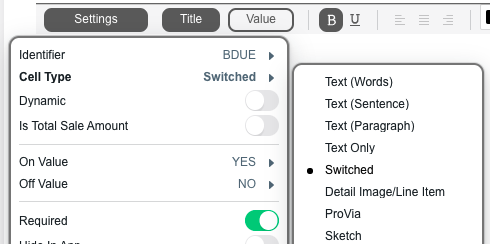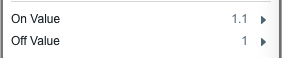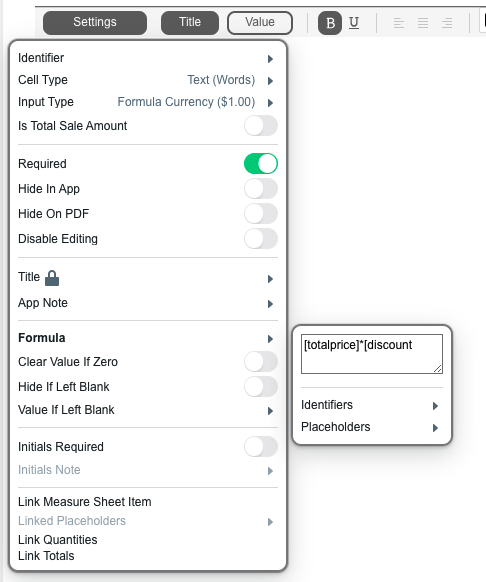# Discount Switches

Because formulas are not able to be used with dynamic cells, we need to use a switch for pricing changes on contracts. The below example is adding a 10% additional fee.
3. Change the cell type to “Switched.”4. Under Settings, set the “On” value to 1.1 and the “Off Value” to 1. 1.1 is the value we will multiply the total by to get the 10% increase. If you want a 25% increase, you would set the “On” value to 1.25 for example.8. Click settings then formula, and multiply the identifier set up in step 5 (for example distance) by the identifier for total price. This should already exist within your contract. For example [totalprice]*[discount].